# 10.1. What are strings?#

In C programming language, there is no data type that stores a word/sentence. Instead, we use strings to store a word or sentence. We did see strings before when we used to print sentences and words using printf. For example, in the printf statement below, "Hello world!\n" is a string.

printf("Hello world!\n");


Notice that words/sentences are enclosed between double-quotes: "<words/sentences>".

## 10.1.1. Store a String#

To store, a string in a variable, we use an array of characters that is “null-terminated”. This means that the string is stored in a special array of characters that stores the word/s, and the last character is a null character. A null character is \0. It has an ASCII code of $$0$$. This means that \0 is encoded using ASCII code to be stored in the main memory as $$0$$. You may refer to Chapter 2: Section 2.2.3 to refresh your memory on ASCII code.

There are three ways we can set up a string in a null-terminated array of characters.

### 10.1.1.1. Method 1: Declare and Initialize#

To declare a 1D array of characters, and initialize its elements to 'H', 'e', 'l', 'l', 'e' and finally a '\0', we can do the following.

char myString[] = "Hello";


The statement will make myString array have $$6$$ elements, where the first $$5$$ elements store the characters in "Hello", and the last element stores '\0' as shown in the figure.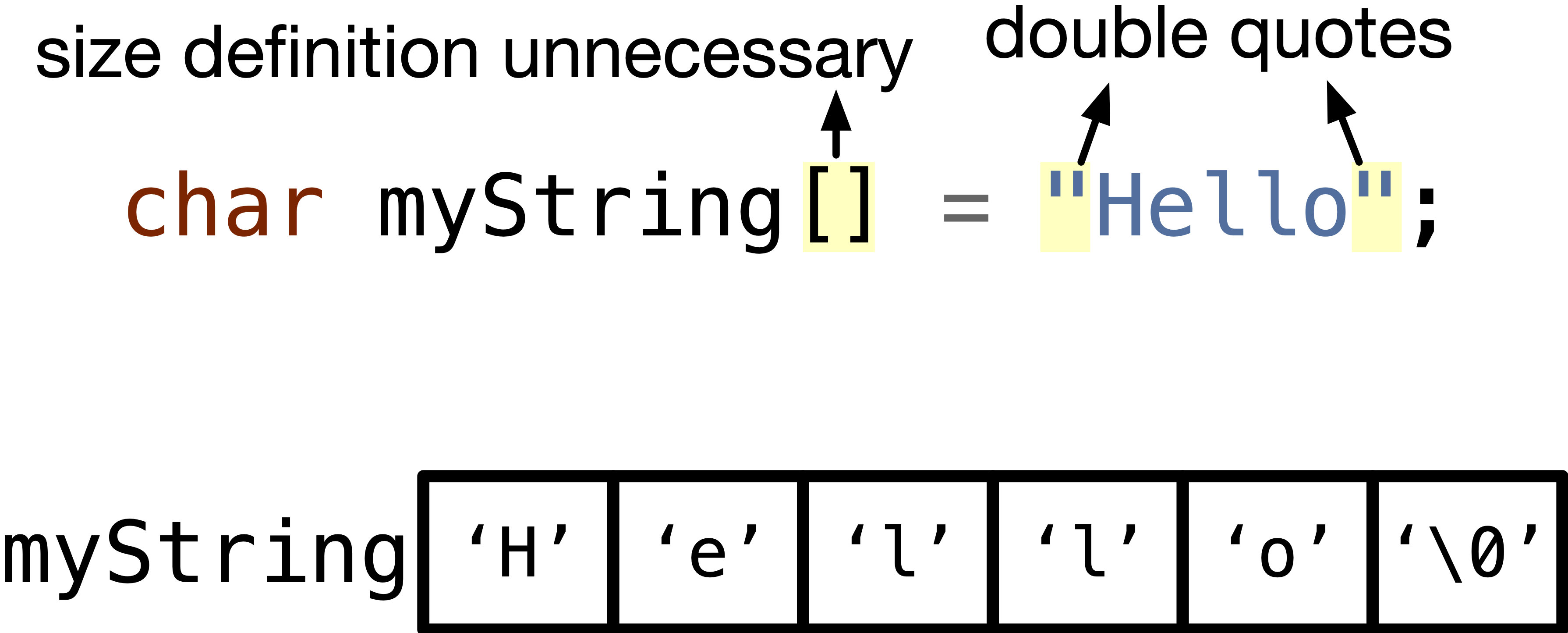Fig. 10.1 Declare and initialize a null-terminated array of characters — a string.#

Although it is unnecessary to define the size of the 1D array when we declare and initialize it, we can still define the size as in the following statement.

char myString[5 + 1] = "Hello";


Notice here that we defined a size of $$6$$ elements although "Hello" has $$5$$ characters. This is because we want to leave an extra element for the null character.

Note

We can have the size of the array to be greater than the number of characters $$+1$$, for example $$8$$ in the following statement,

char myString = "Hello";


This will set the extra two characters to null '\0' as shown in the figure below.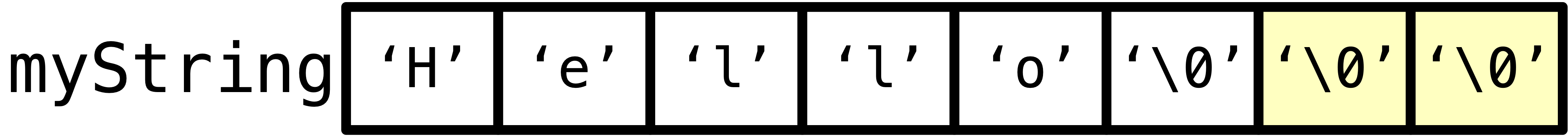Fig. 10.2 Extra elements are set to '\0' at the time of declaration and initialization of a null-terminated array of characters.#

Important

Like any 1D array, you can change individual elements in a null-terminated array. For example,

char myString[] = "Hello";
myString = 'h';


### 10.1.1.2. Method 2: Declare now and Initialize later#

You may declare a 1D array of characters, then change it to a string later in other statements. For example, we can create an array of 4 characters. Then, change this array to store the string "The" as shown in the following statements.

char myString;
myString = 'T';
myString = 'h';
myString = 'e';
myString = '\0';


### 10.1.1.3. Method 3: Declare a Pointer Pointing to a Const String#

We can declare a pointer of type (char*) and initialize it to point to the first character of a “constant string” as in the following statement.

char* pStr = "Wow";


This would create a variable named pStr that can hold an address to a character. This same statement creates an array of characters having “Wow” terminated by null in the constants and global variables memory segment. We are also setting the address stored in pStr to be the address of the first character of the string “Wow” as shown in the figure below.

Cannot Change Individual Elements

You cannot change individual elements in a const string. For example, you cannot do the following:

pStr = 'R';


This is because "Wow" string is stored as a constant, and you cannot change a constant. If you try doing it, your program may fail silently, which means the statement will fail and other statements in your program may not behave as expected, or give a “segmentation fault” error message, which means you are accessing or changing a memory space you are not permitted to access or change.

Can change the value of the pointer

However, pStr is not a constant, and hence you can always change what pStr points to and make it point to another string, like pStr = "Cat";.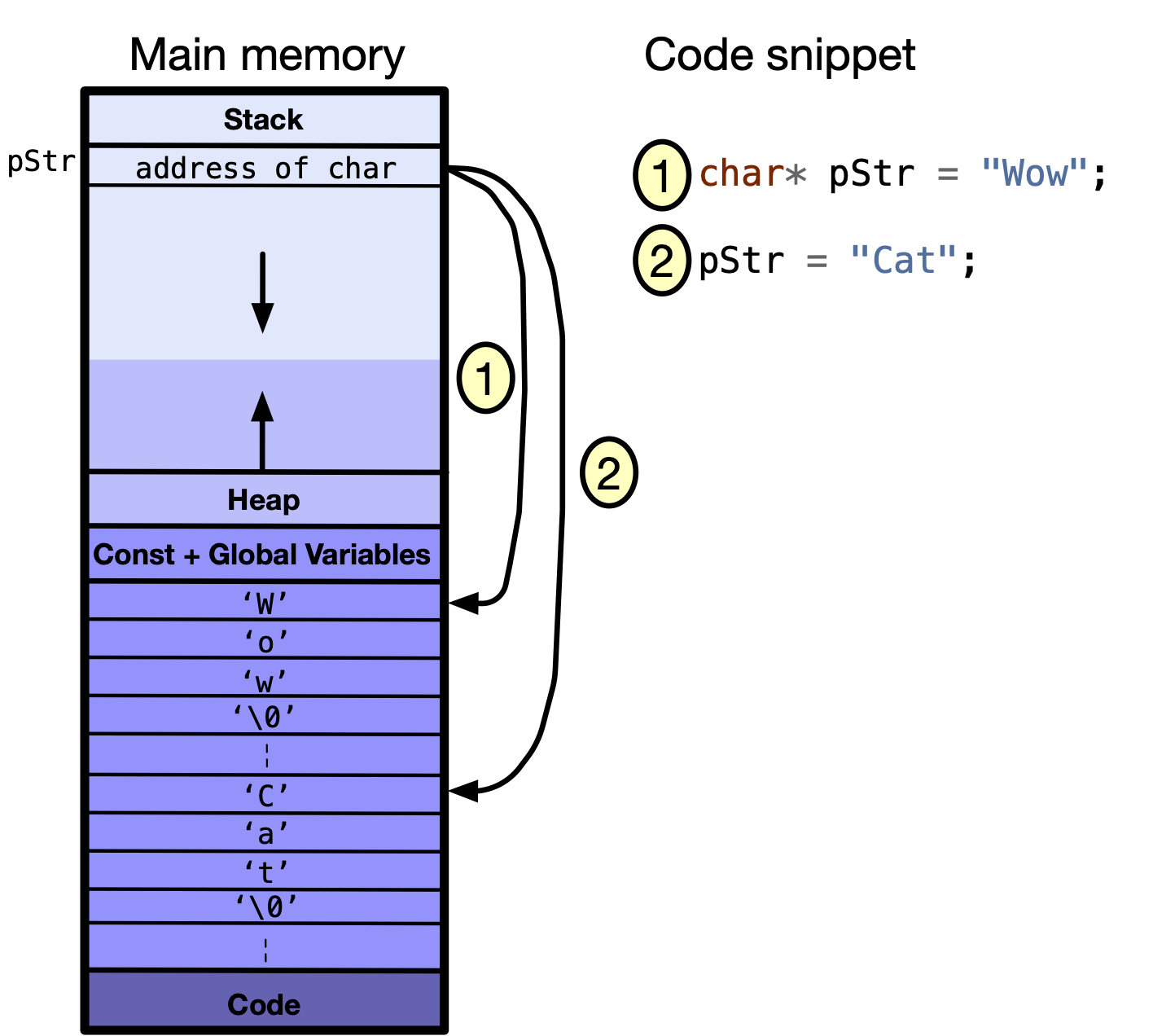Fig. 10.5 Change the value of pStr pointer to have the address of the first character of another constant string.#

Since pStr is not a constant, it can point to the first character in an array of null-terminated characters, i.e. string, on the stack. Recall that the stack is a segment of the memory used to store local variables, which are not constants. For example, the following figure shows how the three statements are executed in the memory.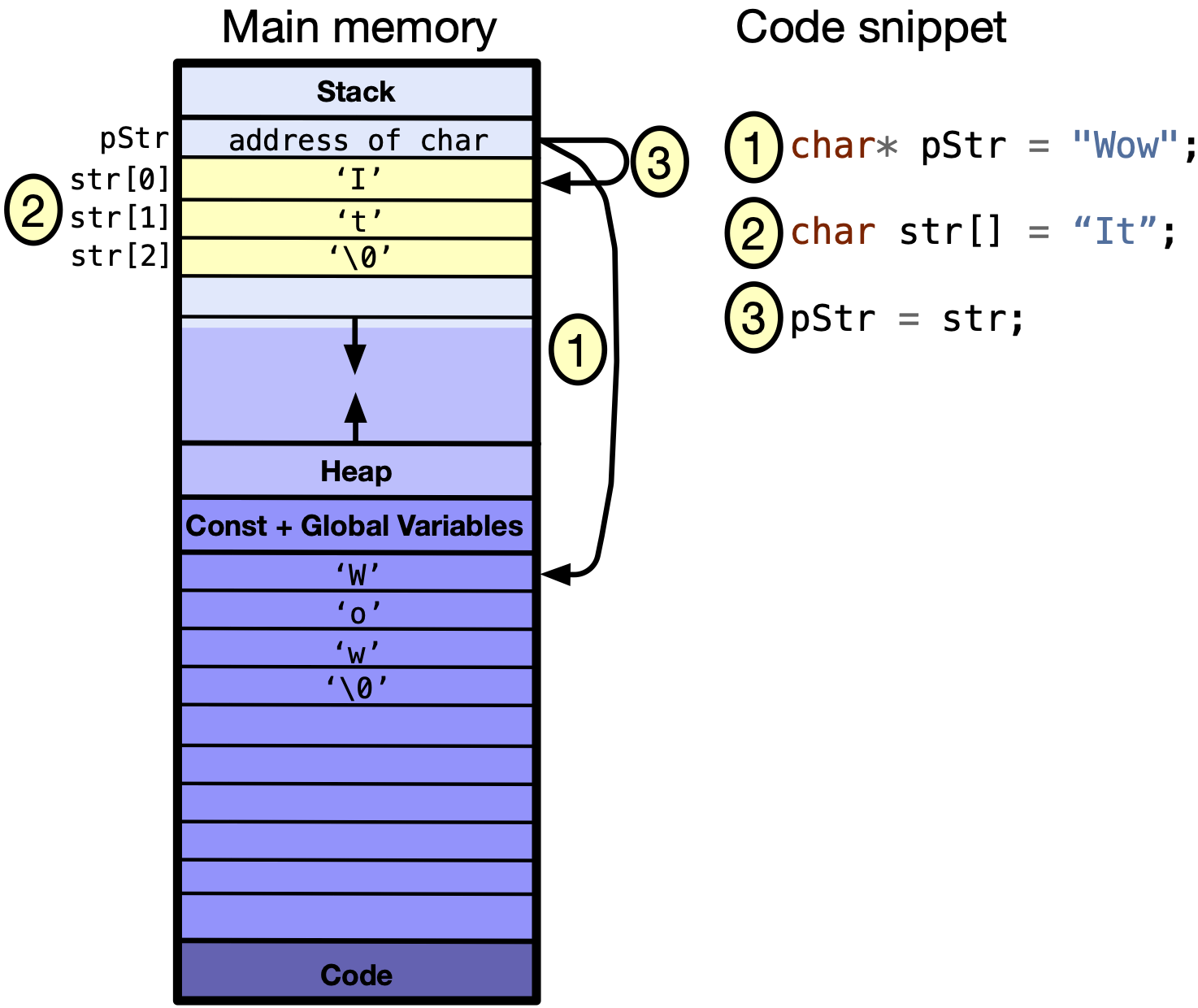Fig. 10.6 Change the value of pStr pointer to have the address of the first character of another string that is on the stack.#

Can access/change characters on the stack

If pStr is now pointing to an array on the stack, the elements of the array can be changed like any other array. For example,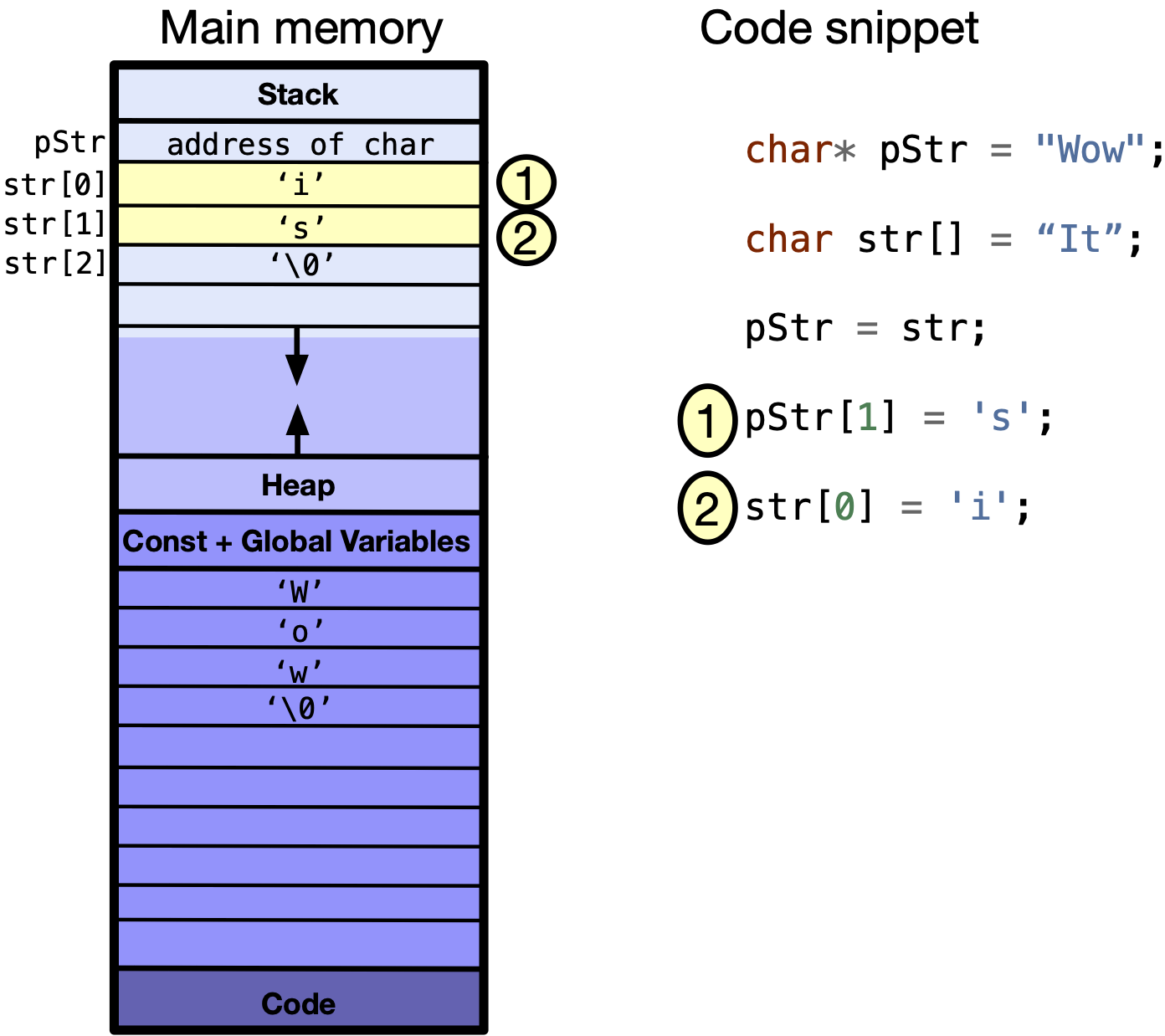Fig. 10.7 You can change the characters on stack pointed to by pStr.#

Cannot change the array identifier

Although you can change what a pointer points to, e.g. by assigning pStr an address of different characters in previous examples, you cannot change an array identifier.

For example, str in char str[] = "Hello"; is an array identifier and a pointer to the first character in the "Hello" string, but str itself is not assignable. For example, if you try compiling the following code, you would get a compile-time error: "array type 'char' is not assignable".

int main(void) {
char str[] = "Hello";
str = "APS105";
return 0;
}


## 10.1.2. What is the usage of the '\0' in a string?#

The null character \0 can be used to know the end of a string, if the size is unknown. It is very handy, whenever we pass strings to functions. For example, let’s write a function to count the number of spaces ' ' in a string.

To pass a string to a function, we use the same syntax as 1D arrays. A string or 1D array can be received as either as char* or char[], for example,

int spacesCount(char str[]);


or

int spacesCount(char* str);


Inside the function, we should check the elements at each index: from 0 to the index that has the last element. The last element here is the element having '\0'. If an element is a space, we increment a counter than counts the number of spaces. We can write the code as follows, or you can download spacesCount.c if you want to run the program yourself.

Code

#include <stdio.h>
#include <string.h>

int spacesCount(char str[]);

int main(void) {
char str[] = "Welcome to Chapter 10 in Snefru";
printf("The number of spaces is %d.\n", spacesCount(str));

return 0;
}

int spacesCount(char str[]) {
int count = 0;
for (int ind = 0; str[ind] != '\0'; ind++) {
if (str[ind] == ' ') {
count++;
}
}
return count;
}


Output

The number of spaces is 5.